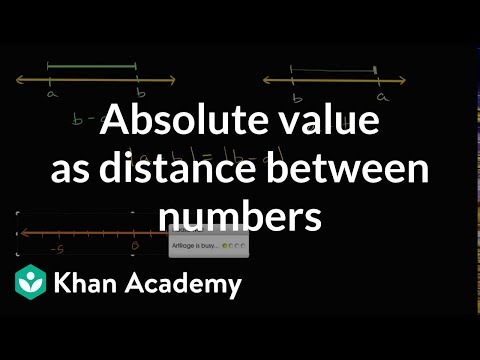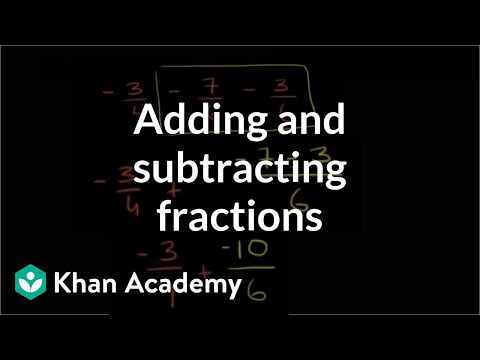Video

# Ordering expressions (Full video)

Description: Let's get some practice thinking about adding and subtracting variables representing positive and negative numbers on the number line. Let's get some practice understanding the variables and the negative numbers that they might represent or the positive numbers. So we're told to order the following expressions by their values from least to greatest. So just to get our bearings, let's see, three hash marks to the left of zero is negative three.

### Other videos you might be interested in### Absolute value as distance between numbers (Full video)